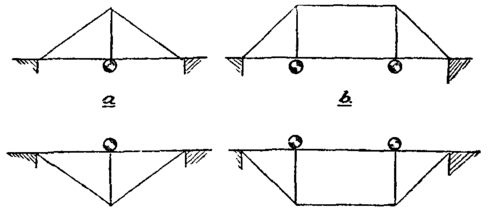Fig. 61.Fig. 62.

Most braced girders may be considered as built up of two simple forms of truss, the king-post truss (fig. 61, a), or the queen-post truss (fig. 61, b). These may be used in either the upright or the inverted position. A multiple truss consists of a number of simple trusses, e.g. Bollman truss. Some timber bridges consist of queen-post trusses in the upright position, as shown diagrammatically in fig. 62, where the circles indicate points at which the flooring girders transmit load to the main girders. Compound trusses consist of simple trusses used as primary, secondary and tertiary trusses, the secondary supported on the primary, and the tertiary on the secondary. Thus, the Fink truss consists of king-post trusses; the Pratt truss (fig. 63) and the Whipple truss (fig. 64) of queen-post trusses alternately upright and inverted.Fig. 64.Fig. 63.

A combination bridge is built partly of timber, partly of steel, the compression members being generally of timber and the tension members of steel. On the Pacific coast, where excellent timber is obtainable and steel works are distant, combination bridges are still largely used (Ottewell, Trans. Am. Soc. C.E. xxvii. p. 467). The combination bridge at Roseburgh, Oregon, is a cantilever bridge, The shore arms are 147 ft. span, the river arms 105 ft., and the suspended girder 80 ft., the total distance between anchor piers being 584 ft. The floor beams, floor and railing are of timber. The compression members are of timber, except the struts and bottom chord panels next the river piers, which are of steel. The tension members are of iron and the pins of steel. The chord blocks and post shoes are of cast-iron.Fig. 65.

33. Graphic Method of finding the Stresses in Braced Structures. - Fig. 65 shows a common form of bridge truss known as a Warren girder, with lines indicating external forces applied to the joints; half the load carried between the two lower joints next the piers on either side is directly carried by the abutments. The sum of the two upward vertical reactions must clearly be equal to the sum of the loads. The lines in the diagram represent the directions of a series of forces which must all be in equilibrium; these lines may, for an object to be explained in the next paragraph, be conveniently named by the letters in the spaces which they separate instead of by the method usually employed in geometry. Thus we shall call the first inclined line on the left hand the line AG, the line representing the first force on the top left-hand joint AB, the first horizontal member at the top left hand the line BH, &C; similarly each point requires at least three letters to denote it; the top first left-hand joint may be called ABHG, being the point where these four spaces meet.

In this method of lettering, every enclosed space must be designated by a letter; all external forces must be represented by lines outside the frame, and each space between any two forces must receive a distinctive letter; this method of lettering was first proposed by O. Henrici and R. H. Bow (Economics of Construction), and is convenient in applying the theory of reciprocal figures to the computation of stresses on frames.

34. Reciprocal Figures. - J. Clerk Maxwell gave (Phil. Mag. 1864) the following definition of reciprocal figures: - "Two plane figures are reciprocal when they consist of an equal number of lines so that corresponding lines in the two figures are parallel, and corresponding lines which converge to a point in one figure form a closed polygon in the other."

Let a frame (without redundant members), and the external forces which keep it in equilibrium, be represented by a diagram constituting one of these two plane figures, then the lines in the other plane figure or the reciprocal will represent in direction and magnitude the forces between the joints of the frame, and, consequently, the stress on each member, as will now be explained.

Reciprocal figures are easily drawn by following definite rules, and afford therefore a simple method of computing the stresses on members of a frame.

The external forces on a frame or bridge in equilibrium under those forces may, by a well-known proposition in statics, be represented by a closed polygon, each side of which is parallel to one force, and represents the force in magnitude as well as in direction. The sides of the polygon may be arranged in any order, provided care is taken so to draw them that in passing round the polygon in one direction this direction may for each side correspond to the direction of the force which it represents.Fig. 66.

This polygon of forces may, by a slight extension of the above definition, be called the reciprocal figure of the external forces, if the sides are arranged in the same order as that of the joints on which they act, so that if the joints and forces be numbered 1, 2, 3, 4, etc., passing round the outside of the frame in one direction, and returning at last to joint 1, then in the polygon the side representing the force 2 will be next the side representing the force 1, and will be followed by the side representing the force 3, and so forth. This polygon falls under the definition of a reciprocal figure given by Clerk Maxwell, if we consider the frame as a point in equilibrium under the external forces.